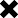### Intrest calculation on savings account

POSTED BY ON April 14, 2012 12:20 am COMMENTS (5)

Hi,

I just want to know how the intrest is calculated on the savings account in a particular bank. I do know that the intrest rate is different in various banks. But what I am not clear is on how its exactly calculated. I heard that its on daily basis. So does it mean its calculated as below:

on Apr1 2012 – 10000 deposited
on Apr2 2012- 20000 deposited
on Apr3 2012- 30000 withdrawn
on Mar31 2013 – 10000 deposited.

Suppose the savings rate is 5% for less than 1 lakh and 6% for more than 1 lakh

The above is an example. So for the above,
Intrest accrued for Apr1 will be : (10000*5%)/365 = Rs. 1.37 (rounding to two decimal)
Intrest accrued for Apr2 will be : (20000*5%)/365= Rs. 2.74
Intrest accrued for Apr 3 is : 0

So the total 1.37+2.74 will be paid on Sept 1 2012 (intrest will be paid on semi monthly basis)

For Mar 31 2013, the total will be 10000+2.74=10002.74
So for this, the intrest will be (10002.74*5%)/365=1.37
and this will be paid on Apr 1 2013

Can somebody confirm if the above calculation is correct?
If not pls let me know how the intrest on savings account is calculated for the above example.

Also, if there are multiple deposits done on a particular day, will the lowest or the highest is considered?
Even in the above example, if the deposit is done on Apr 1st, will it be calculated for Apr 1st or from Apr 2nd?

PK

## 5 replies on this article “Intrest calculation on savings account”

1. Gopal Soni says:

I don’t think the calculation is correct. For the second step the interest should be calculated on 30000 and not on 20000 (total balance on Apr2 2012 is 30000).

Gopal

1. Dear Gopal, Yes you are right for 2nd of april, the end balance is 30K & not 20K.

Thanks

ashal

2. Vaibhav Kini says:

I think they need to consider 365.25, as actually it takes 365.25 i.e 365 and 1/4 day to complete one revolution in a year..

3. Dear PK, in general your calculation is ok. The only thing which I noticed from your example – From 3rd april till 31st march, there was zero balance, the bank may invite penalty on zero balance if not allowed to keep zero balance. 🙂 🙂

Thanks

Ashal

4. Karthik says:

Interest Accruals will be calculated and processed during EOD process in the banks. So the interest will be calculated until the cutoff time for the transactions.
The calculation should use 366 as yr base whenever required. Mostly the banks follow Actual/Actual interest method for accruals and penal interest.

This site uses Akismet to reduce spam. Learn how your comment data is processed.